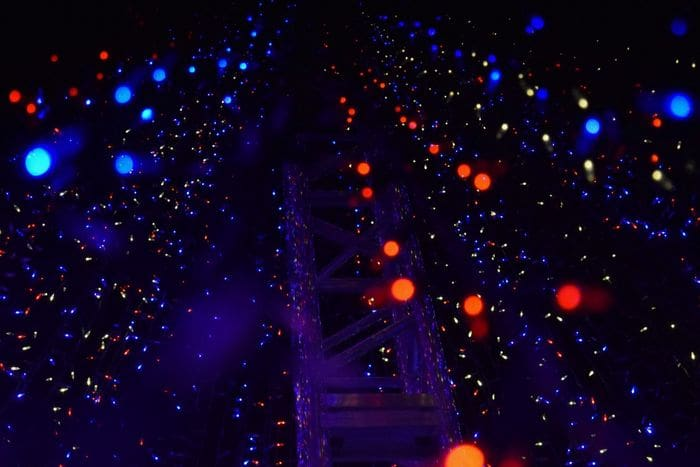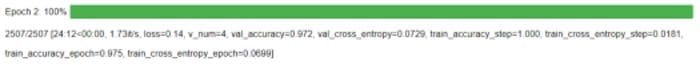By Irfan Alghani Khalid, Computer Science StudentPhoto by Brian Suh on Unsplash

### Introduction

Image classification is a task where we want to predict which class belongs to an image. This task is difficult because of the image representation. If we flatten the image, it will create a long one-dimensional vector. Also, that representation will lose the neighbor information. Therefore, we need deep learning for extracting features and predict the result.

Sometimes, Building a deep learning model can become a difficult task. Although we create a base model for image classification, we need to spend lots of time creating the code. We have to prepare code for preparing the data, training the model, testing the model, and deploy it to the server. And that’s where the Flash comes in!

Flash is a high-level deep learning framework for fast building, training, and testing the deep learning model. Flash is based on the PyTorch framework. So if you know PyTorch, you will be familiar with Flash easily.

In comparison with PyTorch and Lighting, Flash is easy to use but not so flexible as the previous libraries. If you want to build a more complex model, you can use Lightning or straight to the PyTorch.Created by the author.

With Flash, you can build your deep learning model in few lines of code! So, if you are new to deep learning, don’t be afraid. Flash can help you to build a deep learning model without getting confused because of the code.

This article will show you how to build an image classifier using Flash. Without further, let’s get started!

### Install the library

For installing the library, you can use the pip command like this:

``pip install lightning-flash``

If the command doesn’t work, you can install the library by using its GitHub repository. The command looks like this:

After we can download the package successfully, now let’s load the libraries. We also set the seed with the number 42. Here is the code for doing that:

After we install the library, now let’s get the data. For demonstration, we will use the dataset called Cat and Dog dataset.

This dataset contains images that are divided into two classes. The classes are cat and dog. To access the dataset, you can find this dataset at Kaggle. You can access the dataset here.Captured by the author.

After we download the data, now let’s load the dataset into an object. We will use the from_folders method for putting our data into the ImageClassification object. Here is the code for doing that:

After we load the data, the next step is to load the model. Because we will not create our own architecture from scratch, we will use the pre-trained model based on existing convolutional neural network architecture.

We will use the ResNet-50 model that has already pretrained. Also, We set the number of classes based on the dataset. Here is the code for doing that:

### Train the model

After we load the model, now let’s train the model. We need to initialize the Trainer object first. We will train the model in 3 epochs. Also, we enable the GPU to train the model. Here is the code for doing that:

After we initialize the object, now let’s train the model. To train the model, we can use a function called finetune. Inside the function, we set the model and the data. Also, we set the training strategy to freeze, where we don’t want to train the feature extractor. In other words, we train the classifier section only.

Here is the code for doing that:

And here is the evaluation result:Captured by the author.

As you can see from the result, our model has achieved around 97% of accuracy. That’s a good one! Now let’s test the model on several new data.

### Test the model

We will use the sample data that have not been trained on the model. Here are the samples that we will test to the model:

To test the model, we can use the predict method from the flash library. Here is the code for doing that:

As you can see from the result above, the model has predicted the samples with correct labels. That’s nice! Now let’s save the model for later use.

### Save the model

Now we have trained and tested the model. Let’s save the model using the save_checkpoint method. Here is the code for doing that:

If you want to load the model on the other code, you can use the load_from_checkpoint method. Here is the code for doing that:

### Final Remarks

Well done! Now you have learned how to build an image classifier using Flash. As I’ve stated from the beginning, it takes only a few lines of code! How cool is that?

I hope this article can help you to build your own deep learning model on your own case. And I hope you can take a step to learn PyTorch if you want to implement a more complex model.

If you are interested in my article, you can follow me on Medium. I will publish articles related to data science and machine learning. Also, if you have any questions or want to say hi, you can connect with me on LinkedIn.

Thank you for reading my article!

Bio: Irfan Alghani Khalid is a Computer Science Student @ IPB University, interested in Data Science, Machine Learning, and Open Source.

Original. Reposted with permission.

Related: